Type
Quiz
Book Title
Fundamentals of Corporate Finance Standard Edition 9th Edition
ISBN 13
978-0073382395

### 978-0073382395 Chapter 14 Questions and Problems 21-26

April 3, 2019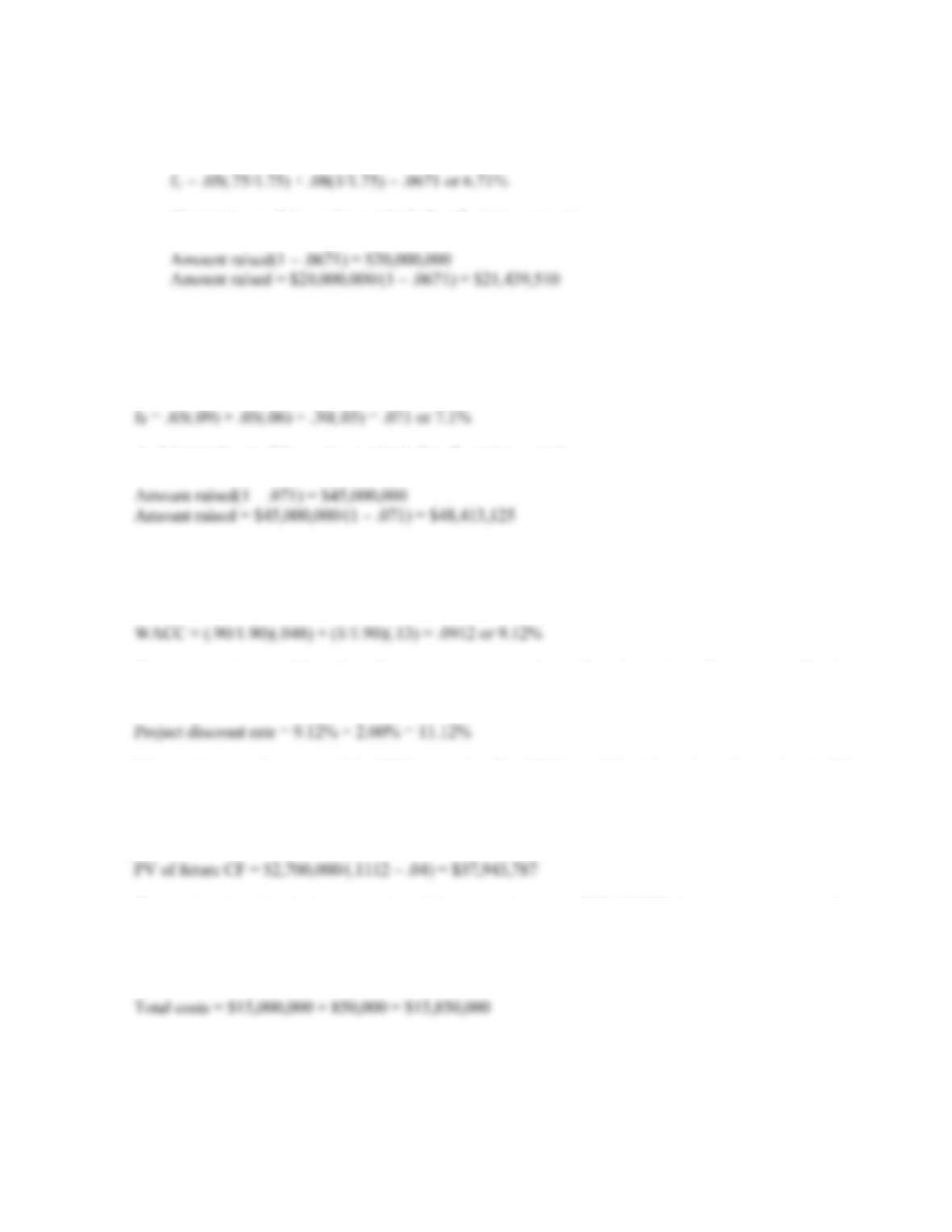CHAPTER 14 B-259
b. The weighted average floatation cost is the weighted average of the floatation costs for debt and
equity, so:
f
c. The total cost of the equipment including floatation costs is:
Even if the specific funds are actually being raised completely from debt, the flotation costs, and
hence true investment cost, should be valued as if the firm’s target capital structure is used.
19. We first need to find the weighted average floatation cost. Doing so, we find:
f
And the total cost of the equipment including floatation costs is:
Intermediate
20. Using the debt-equity ratio to calculate the WACC, we find:
Since the project is riskier than the company, we need to adjust the project discount rate for the
additional risk. Using the subjective risk factor given, we find:
We would accept the project if the NPV is positive. The NPV is the PV of the cash outflows plus the PV
of the cash inflows. Since we have the costs, we just need to find the PV of inflows. The cash inflows
are a growing perpetuity. If you remember, the equation for the PV of a growing perpetuity is the same
as the dividend growth equation, so:
The project should only be undertaken if its cost is less than \$37,943,787 since costs less than this
amount will result in a positive NPV.
21. The total cost of the equipment including floatation costs was: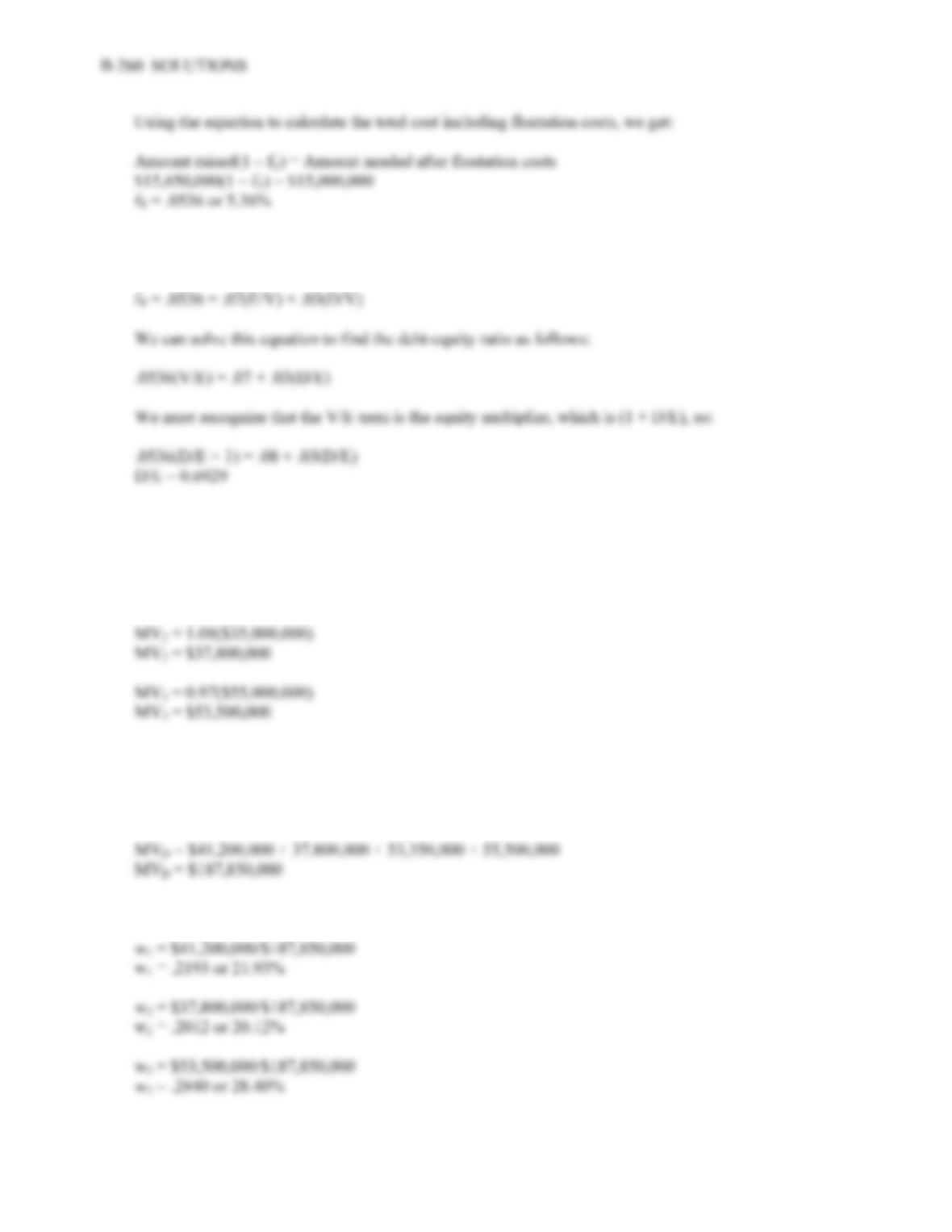Now, we know the weighted average floatation cost. The equation to calculate the percentage floatation
costs is:
f
22. To find the aftertax cost of debt for the company, we need to find the weighted average of the four debt
issues. We will begin by calculating the market value of each debt issue, which is:
MV1 = 1.03(\$40,000,000)
MV1 = \$41,200,000
MV4 = 1.11(\$40,000,000)
MV4 = \$55,500,000
So, the total market value of the company’s debt is:
The weight of each debt issue is:
w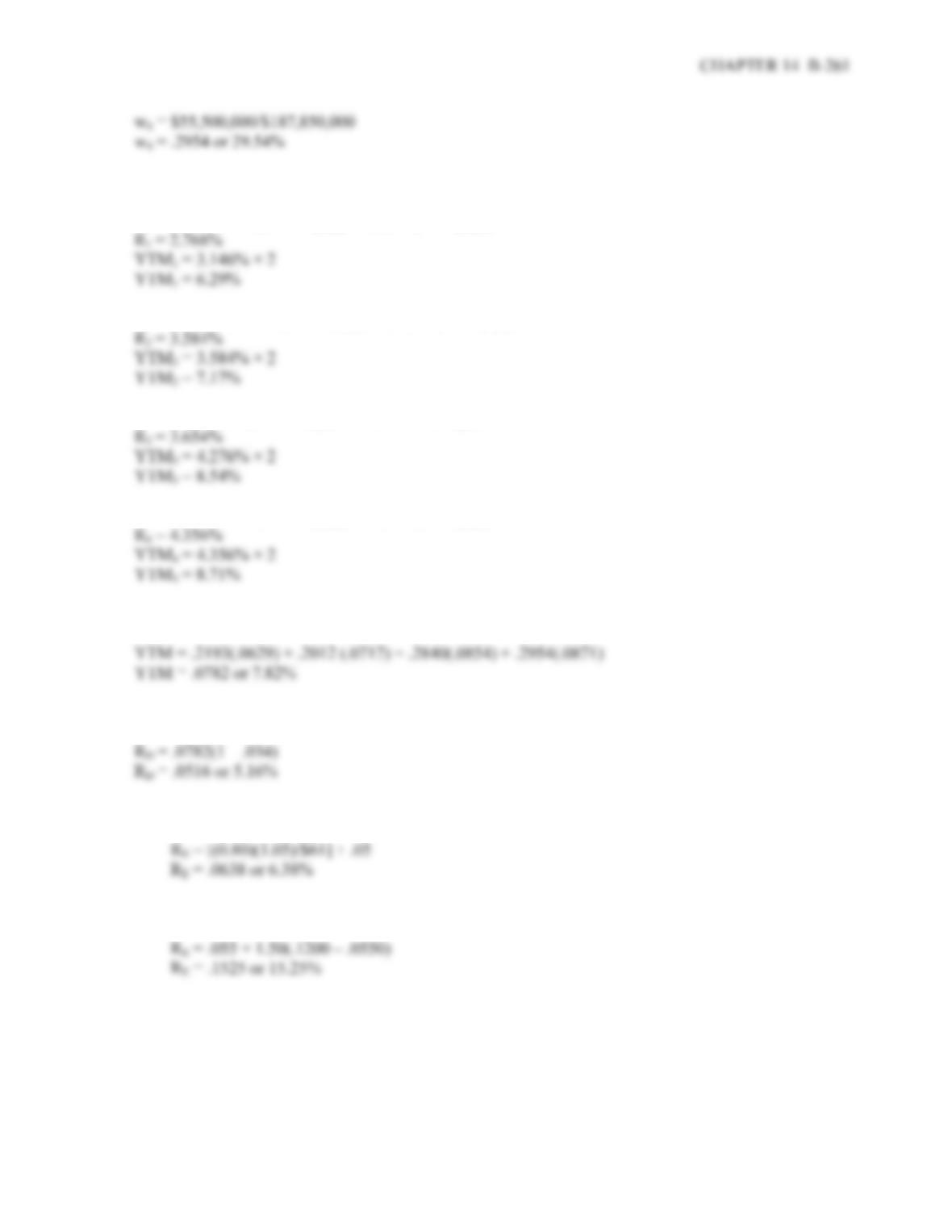Next, we need to find the YTM for each bond issue. The YTM for each issue is:
P
1 = \$1,030 = \$35(PVIFAR%,10) + \$1,000(PVIFR%,10)
R
P
2 = \$1,080 = \$42.50(PVIFAR%,16) + \$1,000(PVIFR%,16)
R
P
3 = \$970 = \$41(PVIFAR%,31) + \$1,000(PVIFR%,31)
R
P
4 = \$1,110 = \$49(PVIFAR%,50) + \$1,000(PVIFR%,50)
R
The weighted average YTM of the company’s debt is thus:
And the aftertax cost of debt is:
R
23. a. Using the dividend discount model, the cost of equity is:
R
b. Using the CAPM, the cost of equity is:
R
c. When using the dividend growth model or the CAPM, you must remember that both are estimates
for the cost of equity. Additionally, and perhaps more importantly, each method of estimating
the cost of equity depends upon different assumptions.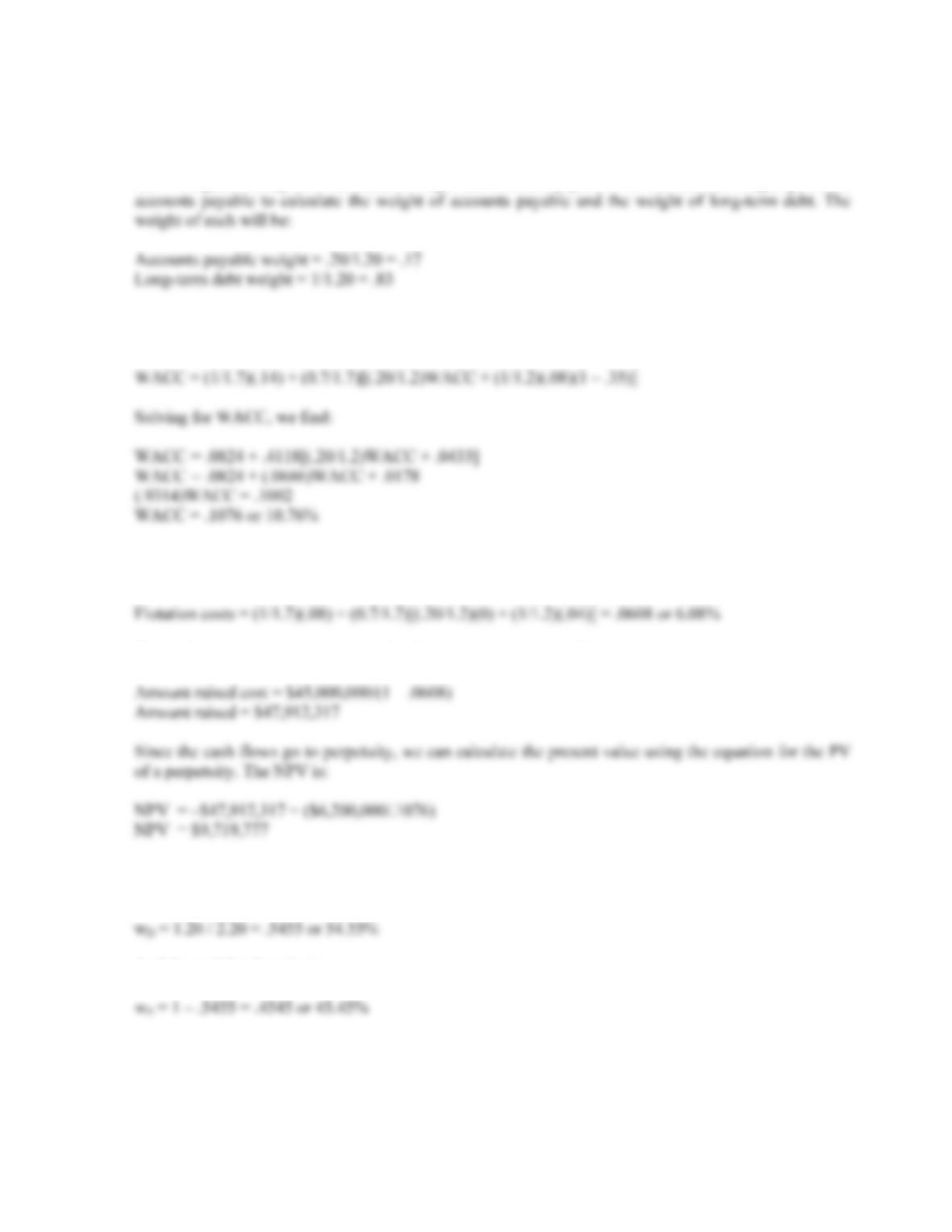B-262 SOLUTIONS
Challenge
24. We can use the debt-equity ratio to calculate the weights of equity and debt. The debt of the company
has a weight for long-term debt and a weight for accounts payable. We can use the weight given for
Since the accounts payable has the same cost as the overall WACC, we can write the equation for the
WACC as:
We will use basically the same equation to calculate the weighted average floatation cost, except we
will use the floatation cost for each form of financing. Doing so, we get:
The total amount we need to raise to fund the new equipment will be:
25. We can use the debt-equity ratio to calculate the weights of equity and debt. The weight of debt in the
capital structure is:
w
And the weight of equity is:
w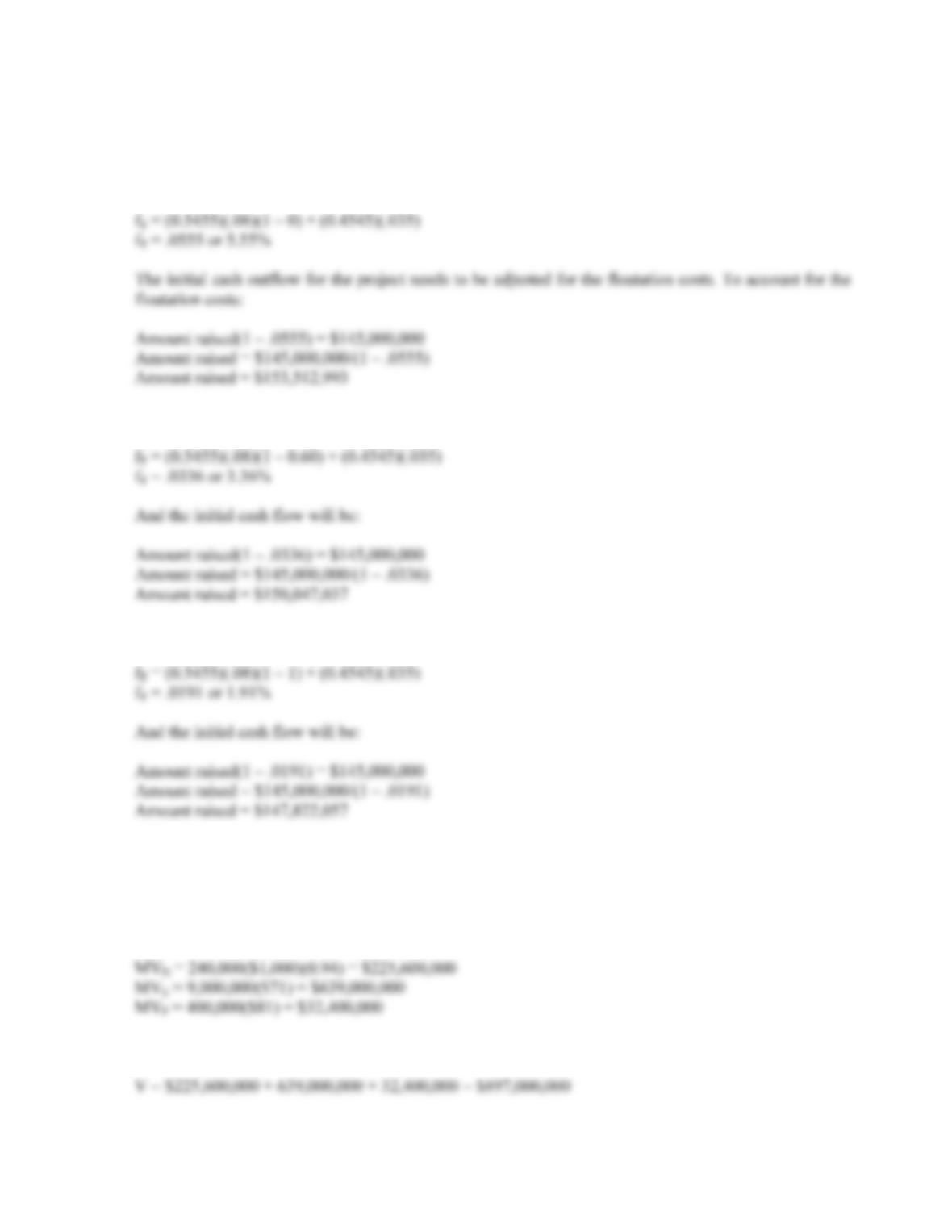CHAPTER 14 B-263
Now we can calculate the weighted average floatation costs for the various percentages of internally
raised equity. To find the portion of equity floatation costs, we can multiply the equity costs by the
percentage of equity raised externally, which is one minus the percentage raised internally. So, if the
company raises all equity externally, the floatation costs are:
f
If the company uses 60 percent internally generated equity, the floatation cost is:
f
If the company uses 100 percent internally generated equity, the floatation cost is:
f
26. The \$4 million cost of the land 3 years ago is a sunk cost and irrelevant; the \$5.1 million appraised
value of the land is an opportunity cost and is relevant. The \$6 million land value in 5 years is a relevant
cash flow as well. The fact that the company is keeping the land rather than selling it is unimportant.
The land is an opportunity cost in 5 years and is a relevant cash flow for this project. The market value
capitalization weights are:
The total market value of the company is: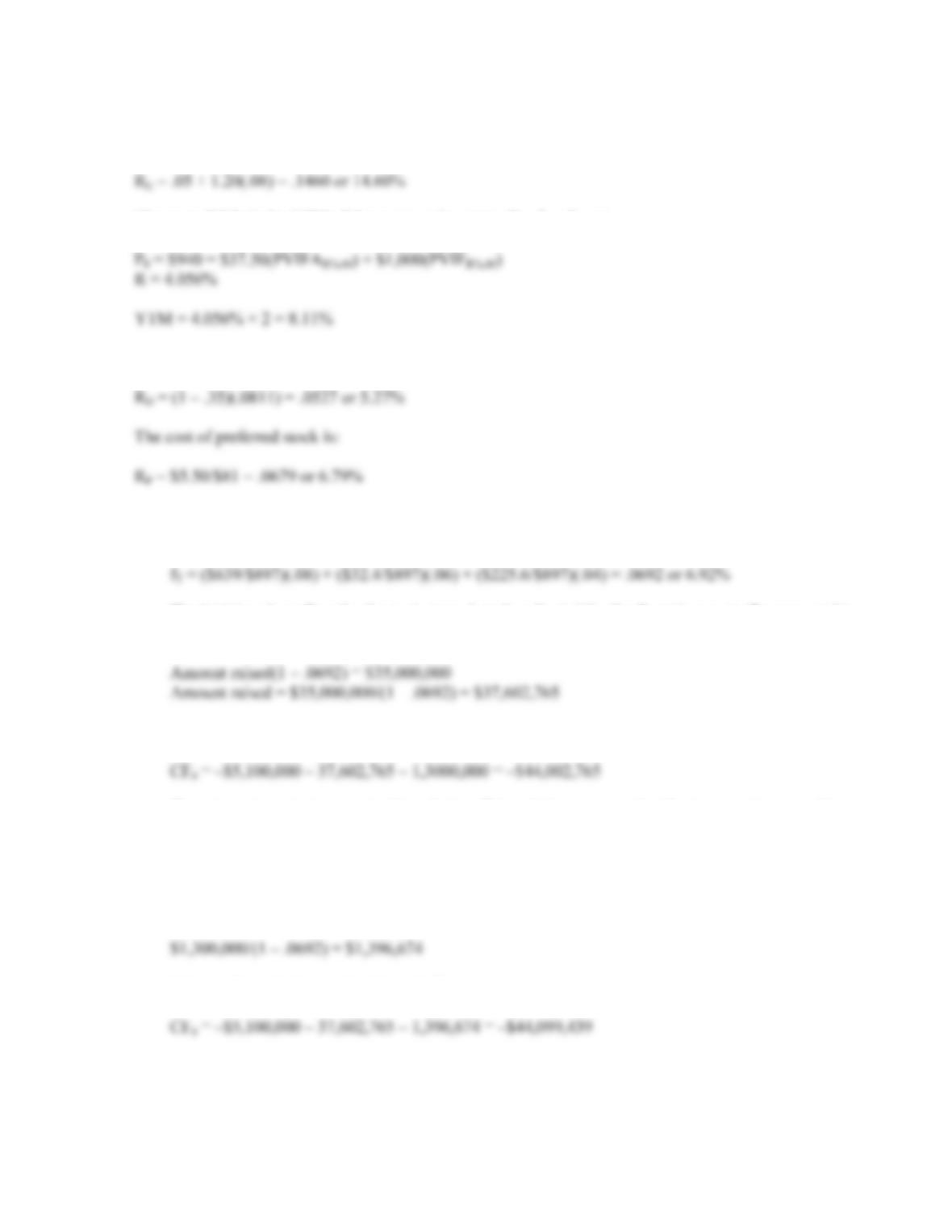B-264 SOLUTIONS
Next we need to find the cost of funds. We have the information available to calculate the cost of equity
using the CAPM, so:
R
The cost of debt is the YTM of the company’s outstanding bonds, so:
P
And the aftertax cost of debt is:
R
a. The weighted average floatation cost is the sum of the weight of each source of funds in the capital
structure of the company times the floatation costs, so:
f
The initial cash outflow for the project needs to be adjusted for the floatation costs. To account for
the floatation costs:
So the cash flow at time zero will be:
There is an important caveat to this solution. This solution assumes that the increase in net working
capital does not require the company to raise outside funds; therefore the floatation costs are not
included. However, this is an assumption and the company could need to raise outside funds for
the NWC. If this is true, the initial cash outlay includes these floatation costs, so:
Total cost of NWC including floatation costs:
This would make the total initial cash flow: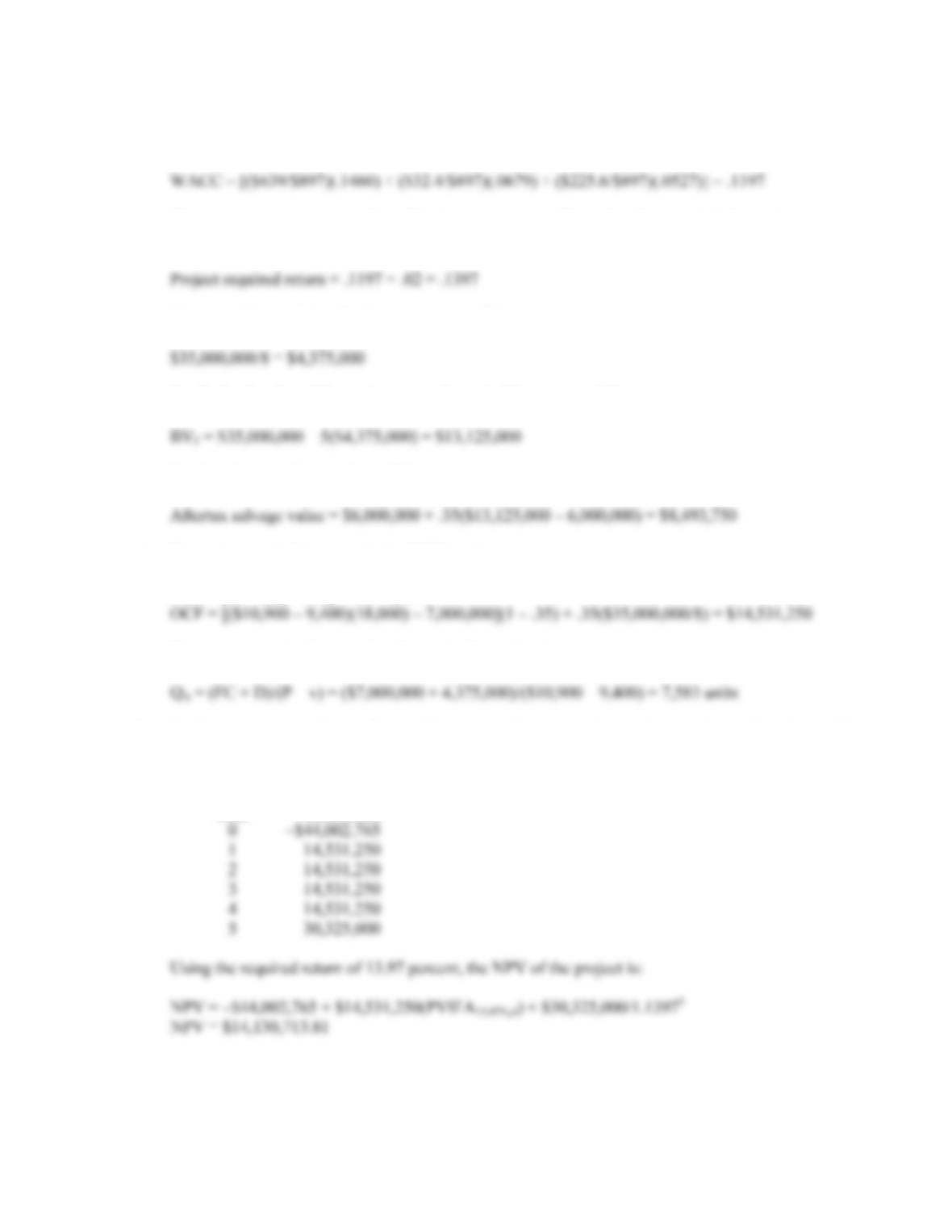CHAPTER 14 B-265
b. To find the required return on this project, we first need to calculate the WACC for the company.
The company’s WACC is:
The company wants to use the subjective approach to this project because it is located overseas.
The adjustment factor is 2 percent, so the required return on this project is:
c. The annual depreciation for the equipment will be:
So, the book value of the equipment at the end of five years will be:
So, the aftertax salvage value will be:
d. Using the tax shield approach, the OCF for this project is:
OCF = [(P – v)Q – FC](1 – t) + tCD
e. The accounting breakeven sales figure for this project is:
f. We have calculated all cash flows of the project. We just need to make sure that in Year 5 we add
back the aftertax salvage value and the recovery of the initial NWC. The cash flows for the project
are:
Year Flow Cash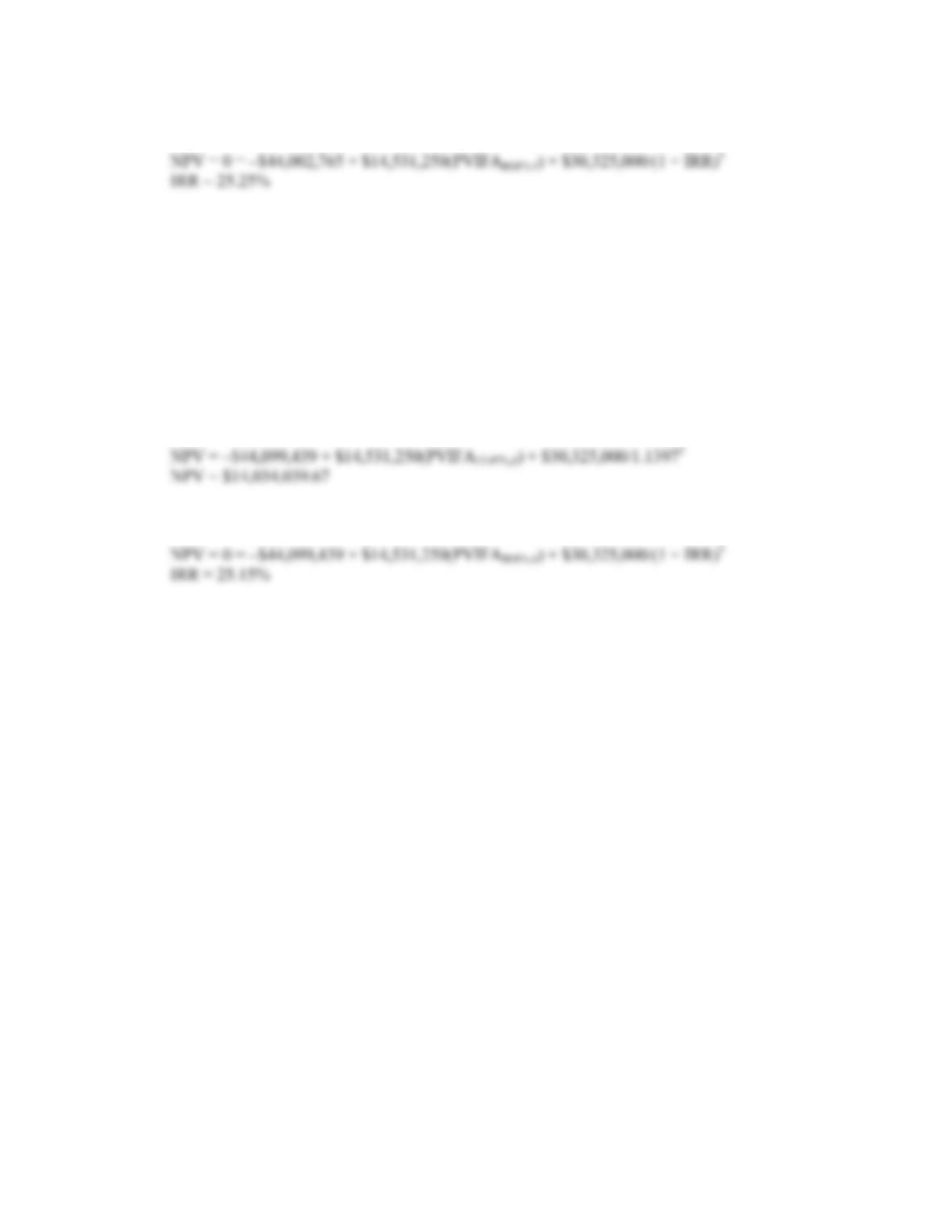B-266 SOLUTIONS
And the IRR is:
If the initial NWC is assumed to be financed from outside sources, the cash flows are:
Year Flow Cash
0 –\$44,099,439
1 14,531,250
2 14,531,250
3 14,531,250
4 14,531,250
5 30,325,000
With this assumption, and the required return of 13.97 percent, the NPV of the project is:
And the IRR is: